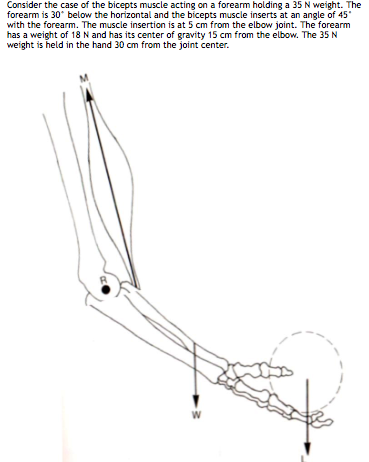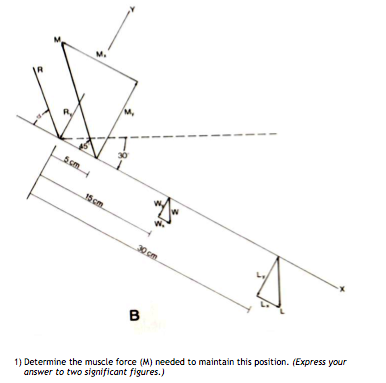# Consider the case of the bicepts muscle acting on a forearm holding a 35 N weight....

###### Question:Consider the case of the bicepts muscle acting on a forearm holding a 35 N weight. The forearm is 30 below the horizontal and the bicepts muscle inserts at an angle of 45 with the forearm. The muscle insertion is at 5 cm from the elbow joint. The forearm has a weight of 18 N and has its center of gravity 15 cm from the elbow. The 35 N weight is held in the hand 30 cm from the joint center.
M. 30 ssm 15cm 30 cm в 1) Determine the muscle force (M) needed to maintain this position. (Express your answer to two significant figures.)

#### Similar Solved Questions

##### Determine the pH during the titration of 26.2 mL of 0.296 M perchloric acid by 0.200...
Determine the pH during the titration of 26.2 mL of 0.296 M perchloric acid by 0.200 M barium hydroxideat the following points: (1) Before the addition of any barium hydroxide (2) After the addition of 9.70 mL of barium hydroxide (3) At the equivalence point (4) After adding 25.2 mL of barium hy...
##### Give an example of a discrete or continuous random variable X (by giving the p.m.f. or...
Give an example of a discrete or continuous random variable X (by giving the p.m.f. or p.d.f.) whose cumulative distribution function F(x) satisfies F(n)=1-1/n! Thank you very much! Exercise 3.40. Give an example of a discrete or continuous random variable X p.d.f.) whose the cumulative distribution...
##### A satellite of mass 230 kg is placed into Earth orbit at a height of 700...
A satellite of mass 230 kg is placed into Earth orbit at a height of 700 km above the surface. (a) Assuming a circular orbit, how long does the satellite take to complete one orbit? (b) What is the satellite's speed? m/s (c) Starting from the satellite on the Earth's surface, what is the min...
##### What is the difference between a root and a factor? for example the number 9
What is the difference between a root and a factor? for example the number 9...
##### A horizontal spring with force constant k = 730 N/m is attached to a wall on...
A horizontal spring with force constant k = 730 N/m is attached to a wall on one end. The other end of the spring is attached to a 1.90 kg object that rests upon a frictionless floor, as shown below. mimi 22 x=0 x= x;/2 i (a) The object is displaced to an initial position of <; = 7.90 cm, extendi...
##### Tion 5 continued) c. Anthracene is known to act as the diene in Diels-Alder read as...
tion 5 continued) c. Anthracene is known to act as the diene in Diels-Alder read as maleic anhydride as shown below. Draw the str reaction and draw a reasonable mechanism illustrating its comma explanation for the observed regioselectivity. he diene in Diels-Alder reactions with dienophiles such s s...
##### The nurse is obtaining preoperative information for a patient who will be having emergency surgery shortly...
The nurse is obtaining preoperative information for a patient who will be having emergency surgery shortly for a ruptured appendix. Which information is crucial for the nurse to assess? Select all that apply....